# Triangle

The figure formed by joining three non collinear points by line segment is called a triangle.
It has three sides, 3 vertex and 3 angle.
The sum of interior angles of triangle is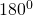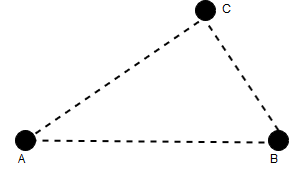.
Exterior angle of triangle– The exterior angle of a triangle is equal to sum of opposite interior angles.
The sum of two side of a triangle is greater than third side.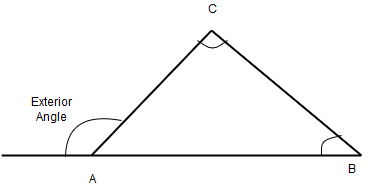Here exterior angle of A=Interior angle of B+Interior angle of C
Between two parallel line and a common base, formed all triangle is equal in area.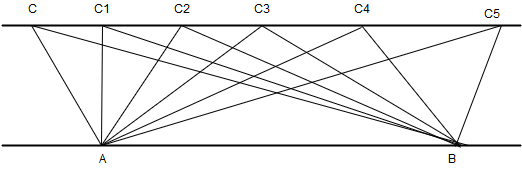In above diagram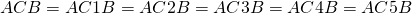Perimeter of triangle– the sum of the length of all side of a triangle is called perimeter of triangle.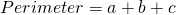Altitude of a triangle– the perpendicular drawn from the vertex of a triangle to the opposite side is called an altitude . all altitude meet at a point.
Median of a triangle– the line joining any vertex of a triangle to the mid-point of its opposite side is called the median of the triangle. That medians meet at a point.
Congruent triangles– two triangles are said to be congruent if every angle of one is equal to corresponding angle of the other and every side of one is equal to the corr esponding side of the other.
Scalene triangle – A triangle whose no side are equal.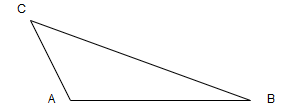Isosceles triangle – A triangle whose any two side are equal to each other is called an isosceles.hereThe opposite angle of equal side is equal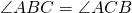Equilateral triangle – A triangle having all side equal to one another is called an equilateral triangle.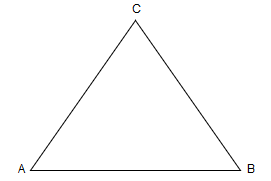In equilateral triangle all angles are equal
if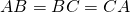thenAcute angle triangle– A triangle in which all the angles are acute angles . Here each angle is less that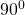Right angle triangle– A triangle whose one angle isis called an right angle triangle.
Obtuse angle triangle– A triangle whose one angle is obtuse more thanis called an obtuse angled triangle.
Area of triangle
1.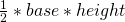2.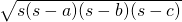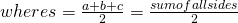Area of equilateral triangle =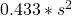where s is side